Want to share your content on R-bloggers? click here if you have a blog, or here if you don't.Photo by Teemu Paananen

# Introduction

As part of my teaching assistant position in a Belgian university, students often ask me for some help in their statistical analyses for their master’s thesis.

A frequent question is how to compare groups of patients in terms of several quantitative continuous variables. Most of us know that:

• To compare two groups, a Student’s t-test should be used1
• To compare three groups or more, an ANOVA should be performed

These two tests are quite basic and have been extensively documented online and in statistical textbooks so the difficulty is not in how to perform these tests.

In the past, I used to do the analyses by following these 3 steps:

1. Draw boxplots illustrating the distributions by group (with the `boxplot()` function or thanks to the `{esquisse}` R Studio addin if I wanted to use the `{ggplot2}` package)
2. Perform a t-test or an ANOVA depending on the number of groups to compare (with the `t.test()` and `oneway.test()` functions for t-test and ANOVA, respectively)
3. Repeat steps 1 and 2 for each variable

This was feasible as long as there were only a couple of variables to test. Nonetheless, most students came to me asking to perform these kind of tests not on one or two variables, but on multiples variables (sometimes up to around 100 variables!). So when there were many variables to test, I quickly realized that I was wasting my time and that there must be a more efficient way to do the job.

# Perform multiple tests at once

I thus wrote a piece of code that automated the process, by drawing boxplots and performing the tests on several variables at once. Below is the code I used, illustrating the process with the `iris` dataset. The `Species` variable has 3 levels, so let’s remove one, and then draw a boxplot and apply a t-test on all 4 continuous variables at once. Note that the continuous variables that we would like to test are variables 1 to 4 in the `iris` dataset.

``````dat <- iris

# remove one level to have only two groups
dat <- subset(dat, Species != "setosa")
dat\$Species <- factor(dat\$Species)

# boxplots and t-tests for the 4 variables at once
for (i in 1:4) { # variables to compare are variables 1 to 4
boxplot(dat[, i] ~ dat\$Species, # draw boxplots by group
ylab = names(dat[i]), # rename y-axis with variable's name
xlab = "Species"
)
print(t.test(dat[, i] ~ dat\$Species)) # print results of t-test
}````````````##
##  Welch Two Sample t-test
##
## data:  dat[, i] by dat\$Species
## t = -5.6292, df = 94.025, p-value = 1.866e-07
## alternative hypothesis: true difference in means is not equal to 0
## 95 percent confidence interval:
##  -0.8819731 -0.4220269
## sample estimates:
## mean in group versicolor  mean in group virginica
##                    5.936                    6.588``````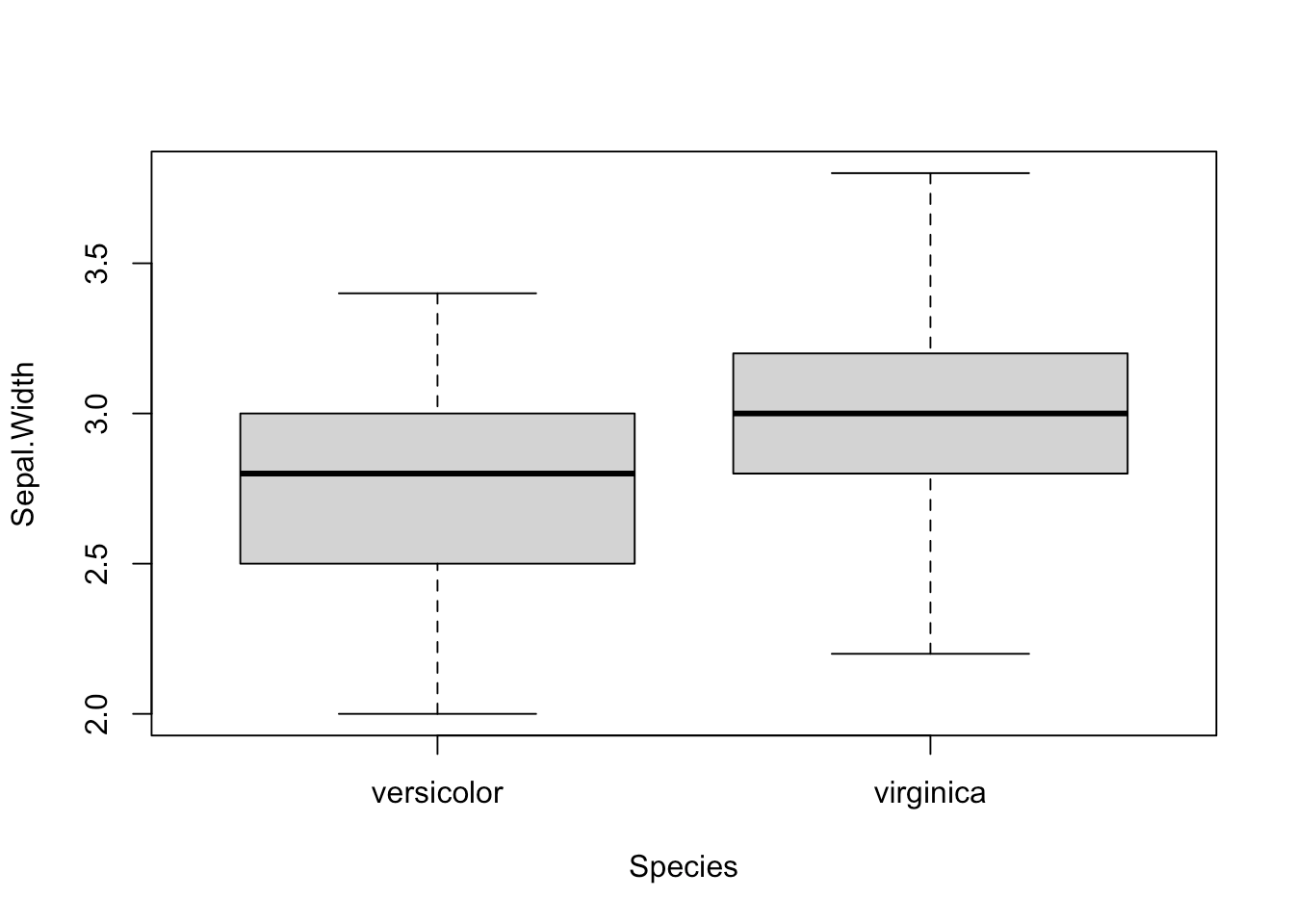``````##
##  Welch Two Sample t-test
##
## data:  dat[, i] by dat\$Species
## t = -3.2058, df = 97.927, p-value = 0.001819
## alternative hypothesis: true difference in means is not equal to 0
## 95 percent confidence interval:
##  -0.33028364 -0.07771636
## sample estimates:
## mean in group versicolor  mean in group virginica
##                    2.770                    2.974````````````##
##  Welch Two Sample t-test
##
## data:  dat[, i] by dat\$Species
## t = -12.604, df = 95.57, p-value < 2.2e-16
## alternative hypothesis: true difference in means is not equal to 0
## 95 percent confidence interval:
##  -1.49549 -1.08851
## sample estimates:
## mean in group versicolor  mean in group virginica
##                    4.260                    5.552``````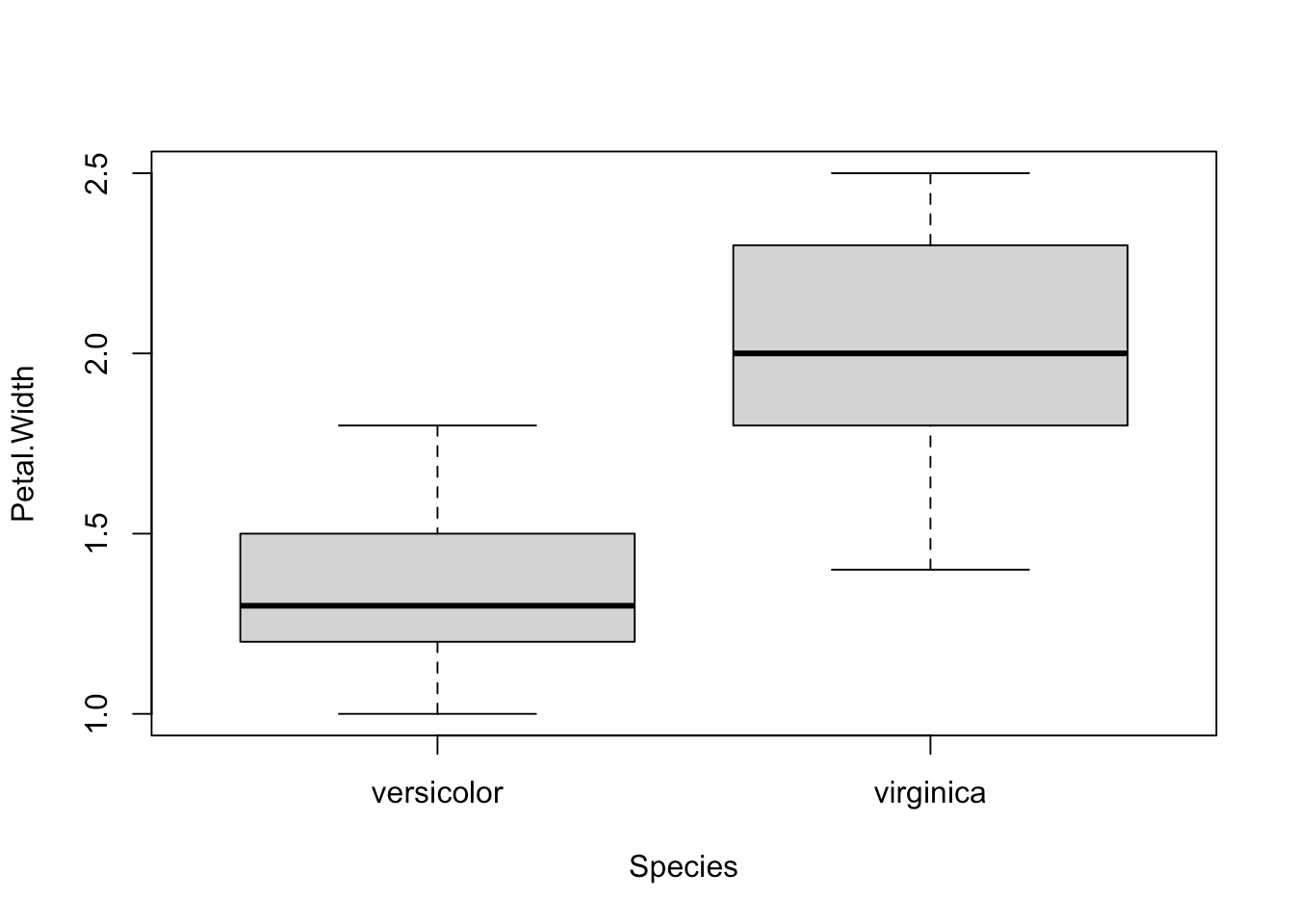``````##
##  Welch Two Sample t-test
##
## data:  dat[, i] by dat\$Species
## t = -14.625, df = 89.043, p-value < 2.2e-16
## alternative hypothesis: true difference in means is not equal to 0
## 95 percent confidence interval:
##  -0.7951002 -0.6048998
## sample estimates:
## mean in group versicolor  mean in group virginica
##                    1.326                    2.026``````

As you can see, the above piece of code draws a boxplot and then prints results of the test for each continuous variable, all at once.

At some point in the past, I even wrote code to:

1. draw a boxplot
2. test for the equality of variances (thanks to the Levene’s test)
3. depending on whether the variances were equal or unequal, the appropriate test was applied: the Welch test if the variances were unequal and the Student’s t-test in the case the variances were equal (see more details about the different versions of the t-test for two samples)
4. apply steps 1 to 3 for all continuous variables at once

I had a similar code for ANOVA in case I needed to compare more than two groups.

The code was doing the job relatively well. Indeed, thanks to this code I was able to test many variables in an automated way in the sense that it compared groups for all variables at once.

The only thing I had to change from one project to another is that I needed to modify the name of the grouping variable and the numbering of the continuous variables to test (`Species` and `1:4` in the above code).

# Concise and easily interpretable results

## T-test

Although it was working quite well and applicable to different projects with only minor changes, I was still unsatisfied with another point.

Someone who is proficient in statistics and R can read and interpret the output of a t-test without any difficulty. However, as you may have noticed with your own statistical projects, most people do not know what to look for in the results and are sometimes a bit confused when they see so many graphs, code, output, results and numeric values in a document. They are quite easily overwhelmed by this mass of information.

With my old R routine, the time I was saving by automating the process of t-tests and ANOVA was (partially) lost when I had to explain R outputs to my students so that they could interpret the results correctly. Although most of the time it simply boiled down to pointing out what to look for in the outputs (i.e., p-values), I was still losing quite a lot of time because these outputs were, in my opinion, too detailed for most real-life applications. In other words, too much information seemed to be confusing for many people so I was still not convinced that it was the most optimal way to share statistical results to nonscientists.

Of course, they came to me for statistical advices, so they expected to have these results and I needed to give them answers to their questions and hypotheses. Nonetheless, I wanted to find a better way to communicate these results to this type of audience, with the minimum of information required to arrive at a conclusion. No more and no less than that.

After a long time spent online trying to figure out a way to present results in a more concise and readable way, I discovered the `{ggpubr}` package. This package allows to indicate the test used and the p-value of the test directly on a ggplot2-based graph. It also facilitates the creation of publication-ready plots for non-advanced statistical audiences.

After many refinements and modifications of the initial code (available in this article), I finally came up with a rather stable and robust process to perform t-tests and ANOVA for many variables at once, and more importantly, make the results concise and easily readable by anyone (statisticians or not).

A graph is worth a thousand words, so here are the exact same tests than in the previous section, but this time with my new R routine:

``````library(ggpubr)

# Edit from here #
x <- which(names(dat) == "Species") # name of grouping variable
y <- which(names(dat) == "Sepal.Length" # names of variables to test
| names(dat) == "Sepal.Width"
| names(dat) == "Petal.Length"
| names(dat) == "Petal.Width")
method <- "t.test" # one of "wilcox.test" or "t.test"
paired <- FALSE # if paired make sure that in the dataframe you have first all individuals at T1, then all individuals again at T2
# Edit until here

# Edit at your own risk
for (i in y) {
for (j in x) {
ifelse(paired == TRUE,
p <- ggpaired(dat,
x = colnames(dat[j]), y = colnames(dat[i]),
color = colnames(dat[j]), line.color = "gray", line.size = 0.4,
palette = "npg",
legend = "none",
xlab = colnames(dat[j]),
ylab = colnames(dat[i]),
),
p <- ggboxplot(dat,
x = colnames(dat[j]), y = colnames(dat[i]),
color = colnames(dat[j]),
palette = "npg",
legend = "none",
)
)
print(p + stat_compare_means(aes(label = paste0(..method.., ", p-value = ", ..p.format.., " (", ifelse(..p.adj.. >= 0.05, "not significant", ..p.signif..), ")")),
method = method,
paired = paired,
# group.by = NULL,
ref.group = NULL
))
}
}``````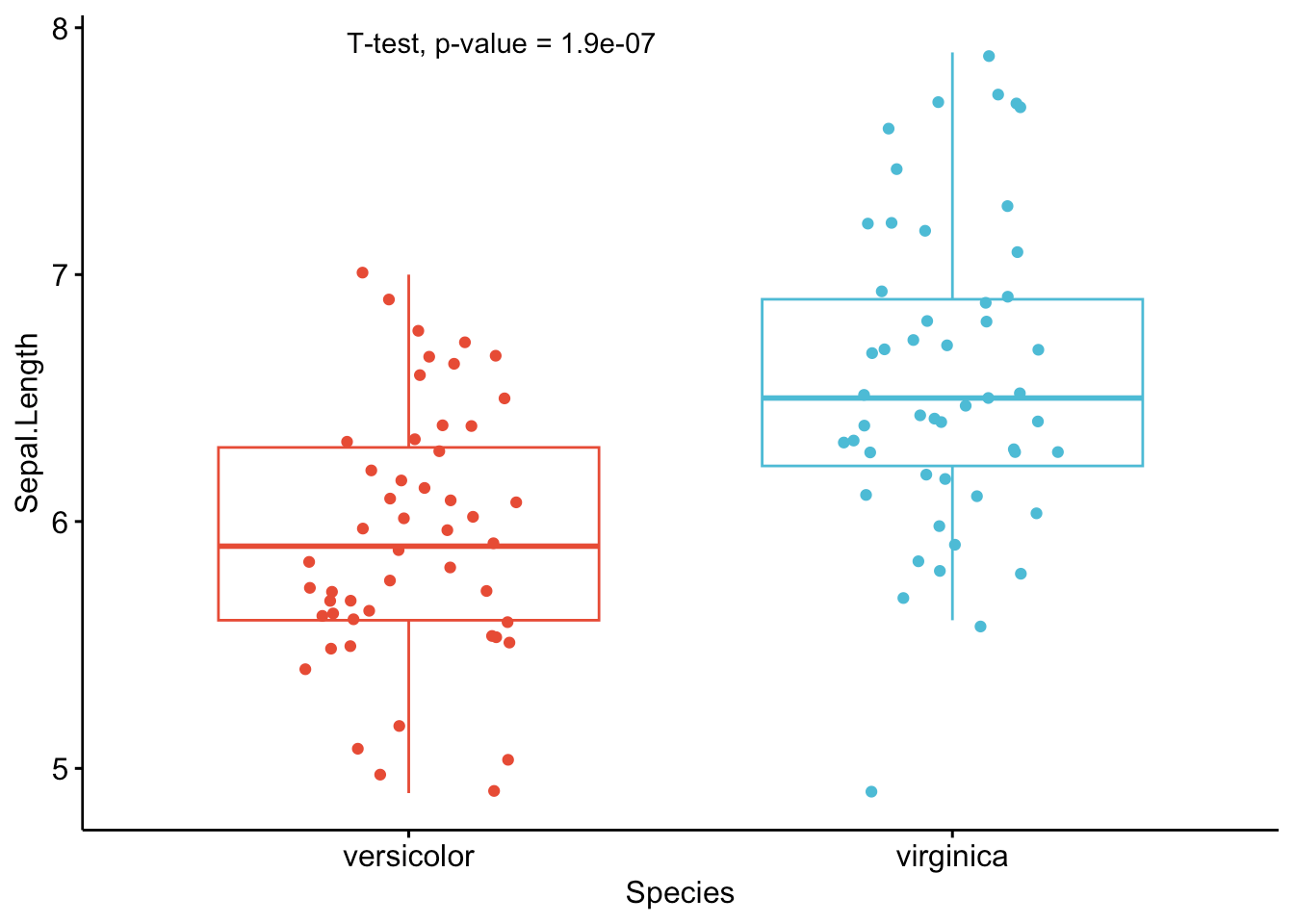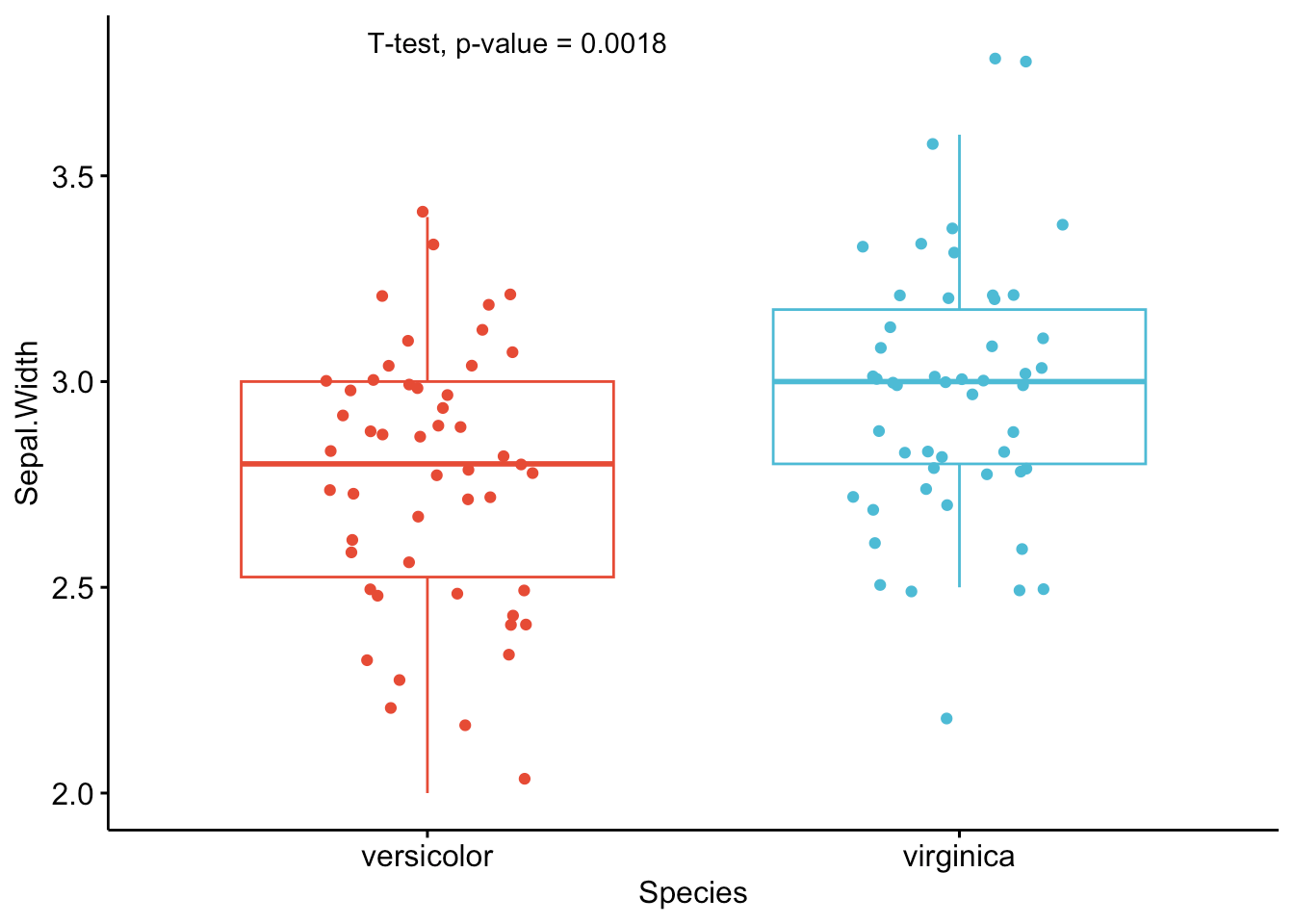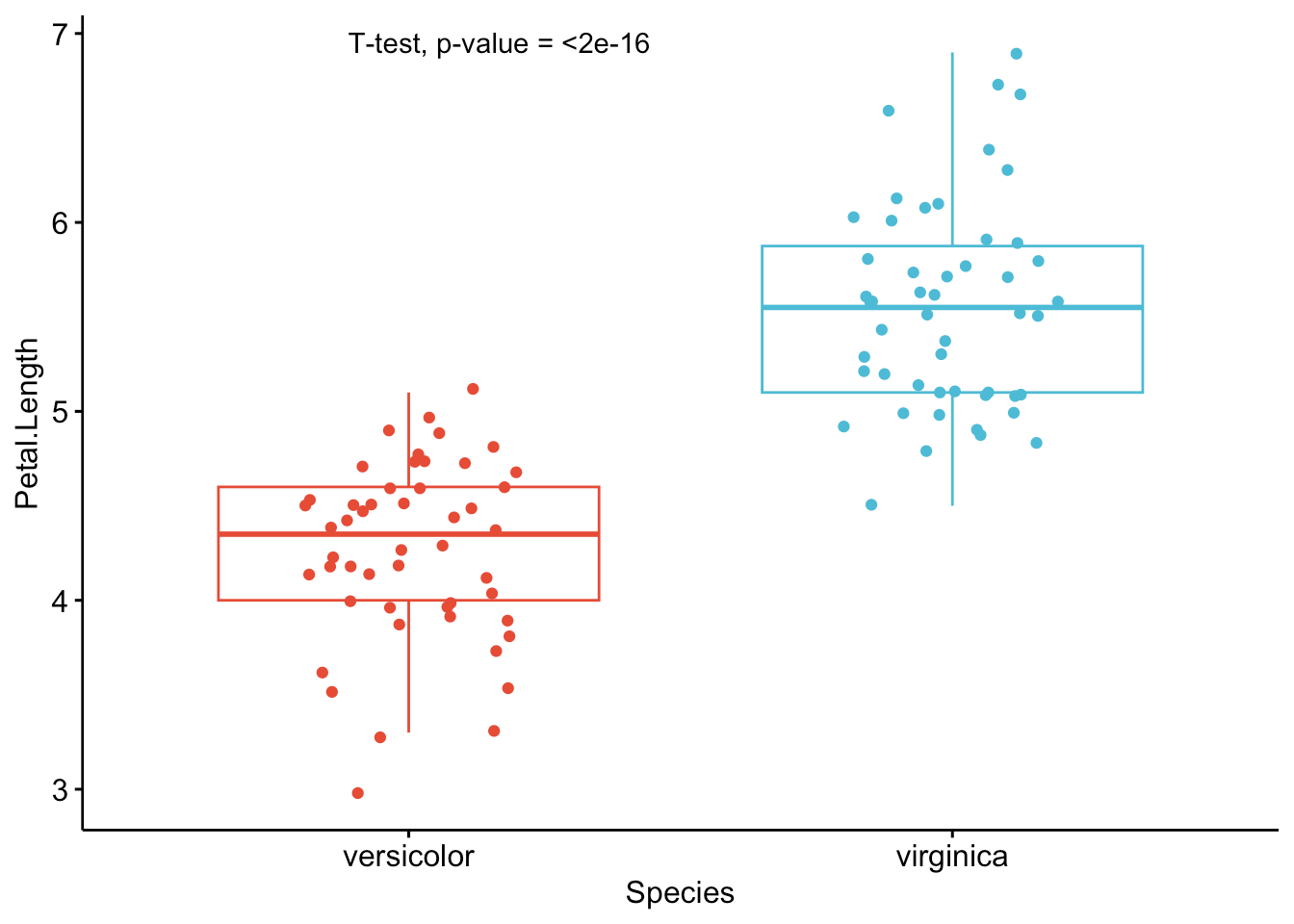As you can see from the graphs above, only the most important information is presented for each variable:

• a visual comparison of the groups thanks to boxplots
• the name of the statistical test
• the p-value of the test

Based on these graphs, it is easy, even for non-experts, to interpret the results and conclude that the `versicolor` and `virginica` species are significantly different in terms of all 4 variables (since p-values < 0.05).

Of course, experts may be interested in more advanced results. However, this simple yet complete graph, which includes the name of the test and the p-value, gives all the necessary information to answer the question: “Are the groups different?”.

In my experience, I have noticed that students and professionals (especially those from a less scientific background) understand way better these results than the ones presented in the previous section.

The only lines of code that need to be modified for your own project is the name of the grouping variable (`Species` in the above code), the names of the variables you want to test (`Sepal.Length`, `Sepal.Width`, etc.),2 whether you want to apply a t-test (`t.test`) or Wilcoxon test (`wilcox.test`) and whether the samples are paired or not (`FALSE` if samples are independent, `TRUE` if they are paired).

## ANOVA

Below the same process with an ANOVA. Note that we reload the dataset `iris` to include all three `Species` this time:

``````dat <- iris

# Edit from here
x <- which(names(dat) == "Species") # name of grouping variable
y <- which(names(dat) == "Sepal.Length" # names of variables to test
| names(dat) == "Sepal.Width"
| names(dat) == "Petal.Length"
| names(dat) == "Petal.Width")
method1 <- "anova" # one of "anova" or "kruskal.test"
method2 <- "t.test" # one of "wilcox.test" or "t.test"
my_comparisons <- list(c("setosa", "versicolor"), c("setosa", "virginica"), c("versicolor", "virginica")) # comparisons for post-hoc tests
# Edit until here

# Edit at your own risk
for (i in y) {
for (j in x) {
p <- ggboxplot(dat,
x = colnames(dat[j]), y = colnames(dat[i]),
color = colnames(dat[j]),
legend = "none",
palette = "npg",
)
print(
p + stat_compare_means(aes(label = paste0(..method.., ", p-value = ", ..p.format.., " (", ifelse(..p.adj.. > 0.05, "not significant", ..p.signif..), ")")),
method = method1, label.y = max(dat[, i], na.rm = TRUE)
)
+ stat_compare_means(comparisons = my_comparisons, method = method2, label = "p.format") # remove if p-value of ANOVA or Kruskal-Wallis test >= 0.05
)
}
}``````Like the improved routine for the t-test, I have noticed that students and non-expert professionals understand ANOVA results presented this way much more easily compared to the default R outputs.

With one graph for each variable, it is easy to see that all species are different from each other in terms of all 4 variables (since all p-values of post-hoc tests < 0.05).

If you want to apply the same automated process to your data, you will need to modify the name of the grouping variable (`Species`), the names of the variables you want to test (`Sepal.Length`, etc.), whether you want to perform an ANOVA (`anova`) or Kruskal-Wallis test (`kruskal.test`) and finally specify the comparisons for the post-hoc tests.3

# To go even further

As we have seen, these two improved R routines allow to:

1. Perform t-tests and ANOVA on a small or large number of variables with only minor changes to the code. I basically only have to replace the variable names and the name of the test I want to use. It takes almost the same time to test one or dozens of variables so it is quite an improvement compared to testing one variable at a time
2. Share test results in a much proper and cleaner way. This is possible thanks to a graph showing the observations by group and the p-value of the appropriate test included on this graph. This is particularily important when communicating results to a wider audience or to people from diverse backgrounds.

However, like most of my R routines, these two pieces of code are still a work in progress. Below are some additional features I have been thinking of and which could be added in the future to make the process of comparing two or more groups even more optimal:

• Add the possibility to select variables by their numbering in the dataframe. For the moment it is only possible to do it via their names. This will allow to automate the process even further because instead of typing all variable names one by one, we could simply type `4:100` (to test variables 4 to 100 for instance).
• When comparing more than two groups, it is only possible to apply an ANOVA or Kruskal-Wallis test at the moment. A major improvement would be to add the possibility to perform a repeated measures ANOVA (i.e., an ANOVA when the samples are dependent). It is currently already possible to do a t-test with two paired samples, but it is not yet possible to do the same with more than two groups.
• Another less important (yet still nice) feature when comparing more than 2 groups would be to automatically apply post-hoc tests only in the case where the null hypothesis of the ANOVA or Kruskal-Wallis test is rejected (so when there is at least one group different from the others, because if the null hypothesis of equal groups is not rejected we do not apply a post-hoc test). At the present time, I manually add or remove the code that displays the p-values of post-hoc tests depending on the global p-value of the ANOVA or Kruskal-Wallis test.

I will try to add these features in the future, or I would be glad to help if the author of the {ggpubr} package needs help in including these features (I hope he will see this article!).

As always, if you have a question or a suggestion related to the topic covered in this article, please add it as a comment so other readers can benefit from the discussion. If you find a mistake or bug, you can inform me by raising an issue on GitHub. For all other requests, you can contact me here.

Related articles:

1. In theory, an ANOVA can also be used to compare two groups as it will give the same results compared to a Student’s t-test, but in practice we use the Student’s t-test to compare two samples and the ANOVA to compare three samples or more.

2. Do not forget to separate the variables you want to test with `|`.

3. Post-hoc test is only the name used to refer to a specific type of statistical tests. Post-hoc test includes, among others, the Tukey HSD test, the Bonferroni correction, Dunnett’s test. Even if an ANOVA or a Kruskal-Wallis test can determine whether there is at least one group that is different from the others, it does not allow us to conclude which are different from each other. For this purpose, there are post-hoc tests that compare all groups two by two to determine which ones are different, after adjusting for multiple comparisons. Concretely, post-hoc tests are performed to each possible pair of groups after an ANOVA or a Kruskal-Wallis test has shown that there is at least one group which is different (hence “post” in the name of this type of test). The null and alternative hypotheses and the interpretations of these tests are similar to a Student’s t-test for two samples.